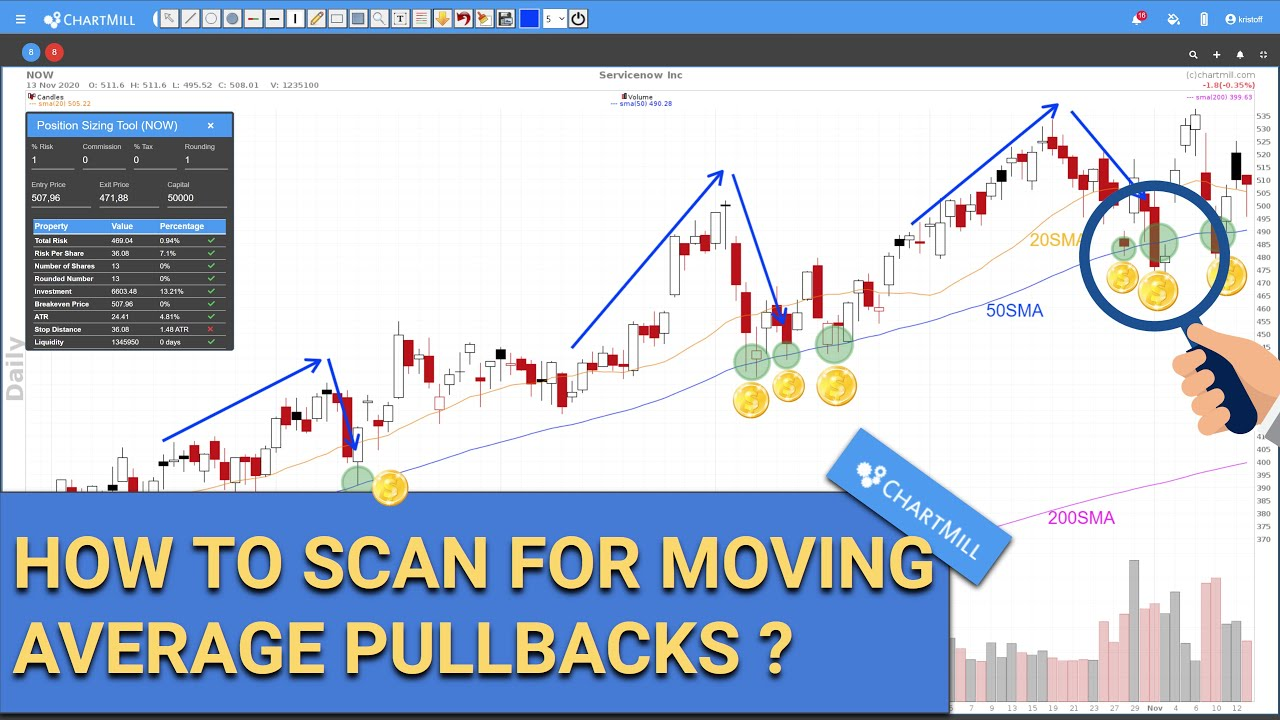# The Simple Moving Average (SMA)

In this first article I will discuss the Simple Moving Average. This is one of the three different types of moving averages available in the ChartMill stock screener, which are :

## First, what exactly is a moving average?

The moving average (MA) is a technical indicator that accumulates price points of an instrument over a given period of time and divides them by the number of points, creating a single trend line. The MA is popular among investors because it helps determine the direction of the current trend and reduces the influence of random peaks.

Calculating an MA requires a certain, sometimes considerable amount of data, depending on the chosen length of the moving average. A 10-day MA requires data of 10 days, whereas an MA over a period of one year requires data of 365 days.

The indicator is called 'moving' because new data replace the oldest data and thus allow the line in the graph to move forward.## The Simple Moving Average (SMA) - Definition and Formula

The SMA is the most basic type and simply calculates the average price of a set of prices over a period of time. If you want to calculate the SMA over a period of 10 days, take the values of the last 10 days and divide the result by 10.

Suppose the last ten closing prices of a stock are as follows: \$74, \$81, \$92, \$91, \$86, \$87, \$91, \$92, \$103 and \$107. The simple moving average adds these numbers together and divides the outcome by 10, so that the average comes to \$90.4.

### SMA Formulan = Time Period

## How to use the SMA?

The SMA is mainly used as a trend indicator in swing trading, in the medium term it can also be used as a first basic warning for buy or sell setups if the price is above or below this SMA.In the StockCharts menu, click on the plus sign and choose 'select overlay to add', scroll down to the Simple Moving Average.Next, select the 'Look back period' and choose an appropriate color.The ChartMill Team

### Related Articles### Related Screens### Related videos